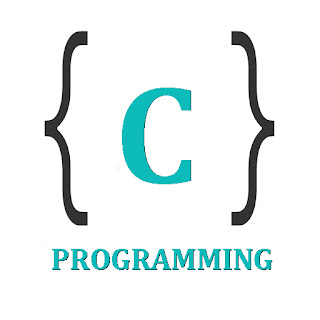# Doubly Linked List C Program Insertion, Deletion and DisplayDoubly Linked List C

### C Program to Implement a Doubly Linked List & provide Insertion, Deletion & Display Operations

#include <stdio.h>
#include <stdlib.h>
struct node
{
struct node *prev;
int n;
struct node *next;
}*h,*temp,*temp1,*temp2,*temp4;
void insert1();
void insert2();
void insert3();
void traversebeg();
void traverseend(int);
void sort();
void search();
void update();
void delete();
int count = 0;
void main()
{
int ch;
h = NULL;
temp = temp1 = NULL;
printf("\n 1 - Insert at beginning");
printf("\n 2 - Insert at end");
printf("\n 3 - Insert at position i");
printf("\n 4 - Delete at i");
printf("\n 5 - Display from beginning");
printf("\n 6 - Display from end");
printf("\n 7 - Search for element");
printf("\n 8 - Sort the list");
printf("\n 9 - Update an element");
printf("\n 10 - Exit");
while (1)
{
printf("\n Enter choice : ");
scanf("%d", &ch);
switch (ch)
{
case 1:
insert1();
break;
case 2:
insert2();
break;
case 3:
insert3();
break;
case 4:
delete();
break;
case 5:
traversebeg();
break;
case 6:
temp2 = h;
if (temp2 == NULL)
printf("\n Error : List empty to display ");
else
{
printf("\n Reverse order of linked list is : ");
traverseend(temp2->n);
}
break;
case 7:
search();
break;
case 8:
sort();
break;
case 9:
update();
break;
case 10:
exit(0);
default:
}
}
}
/* TO create an empty node */
void create()
{
int data;
temp =(struct node *)malloc(1*sizeof(struct node));
temp->prev = NULL;
temp->next = NULL;
printf("\n Enter value to node : ");
scanf("%d", &data);
temp->n = data;
count++;
}
/*  TO insert at beginning */
void insert1()
{
if (h == NULL)
{
create();
h = temp;
temp1 = h;
}
else
{
create();
temp->next = h;
h->prev = temp;
h = temp;
}
}
/* To insert at end */
void insert2()
{
if (h == NULL)
{
create();
h = temp;
temp1 = h;
}
else
{
create();
temp1->next = temp;
temp->prev = temp1;
temp1 = temp;
}
}
/* To insert at any position */
void insert3()
{
int pos, i = 2;
printf("\n Enter position to be inserted : ");
scanf("%d", &pos);
temp2 = h;
if ((pos < 1) || (pos >= count + 1))
{
printf("\n Position out of range to insert");
return;
}
if ((h == NULL) && (pos != 1))
{
printf("\n Empty list cannot insert other than 1st position");
return;
}
if ((h == NULL) && (pos == 1))
{
create();
h = temp;
temp1 = h;
return;
}
else
{
while (i < pos)
{
temp2 = temp2->next;
i++;
}
create();
temp->prev = temp2;
temp->next = temp2->next;
temp2->next->prev = temp;
temp2->next = temp;
}
}
/* To delete an element */
void delete()
{
int i = 1, pos;
printf("\n Enter position to be deleted : ");
scanf("%d", &pos);
temp2 = h;
if ((pos < 1) || (pos >= count + 1))
{
printf("\n Error : Position out of range to delete");
return;
}
if (h == NULL)
{
printf("\n Error : Empty list no elements to delete");
return;
}
else
{
while (i < pos)
{
temp2 = temp2->next;
i++;
}
if (i == 1)
{
if (temp2->next == NULL)
{
printf("Node deleted from list");
free(temp2);
temp2 = h = NULL;
return;
}
}
if (temp2->next == NULL)
{
temp2->prev->next = NULL;
free(temp2);
printf("Node deleted from list");
return;
}
temp2->next->prev = temp2->prev;
if (i != 1)
temp2->prev->next = temp2->next;    /* Might not need this statement if i == 1 check */
if (i == 1)
h = temp2->next;
printf("\n Node deleted");
free(temp2);
}
count--;
}
/* Traverse from beginning */
void traversebeg()
{
temp2 = h;
if (temp2 == NULL)
{
printf("List empty to display \n");
return;
}
printf("\n Linked list elements from begining : ");
while (temp2->next != NULL)
{
printf(" %d ", temp2->n);
temp2 = temp2->next;
}
printf(" %d ", temp2->n);
}
/* To traverse from end recursively */
void traverseend(int i)
{
if (temp2 != NULL)
{
i = temp2->n;
temp2 = temp2->next;
traverseend(i);
printf(" %d ", i);
}
}
/* To search for an element in the list */
void search()
{
int data, count = 0;
temp2 = h;
if (temp2 == NULL)
{
printf("\n Error : List empty to search for data");
return;
}
printf("\n Enter value to search : ");
scanf("%d", &data);
while (temp2 != NULL)
{
if (temp2->n == data)
{
printf("\n Data found in %d position",count + 1);
return;
}
else
temp2 = temp2->next;
count++;
}
}
/* To update a node value in the list */
void update()
{
int data, data1;
printf("\n Enter node data to be updated : ");
scanf("%d", &data);
printf("\n Enter new data : ");
scanf("%d", &data1);
temp2 = h;
if (temp2 == NULL)
{
printf("\n Error : List empty no node to update");
return;
}
while (temp2 != NULL)
{
if (temp2->n == data)
{
temp2->n = data1;
traversebeg();
return;
}
else
temp2 = temp2->next;
}
}
/* To sort the linked list */
void sort()
{
int i, j, x;
temp2 = h;
temp4 = h;
if (temp2 == NULL)
{
printf("\n List empty to sort");
return;
}
for (temp2 = h; temp2 != NULL; temp2 = temp2->next)
{
for (temp4 = temp2->next; temp4 != NULL; temp4 = temp4->next)
{
if (temp2->n > temp4->n)
{
x = temp2->n;
temp2->n = temp4->n;
temp4->n = x;
}
}
}
traversebeg();
}